Note: This addon document is for an older version of GRASS GIS that will be discontinued soon. You should upgrade your GRASS GIS installation, and read the current addon manual page.

## NAME

r.green.hydro.recommended - Calculate hydropower energy potential with user's recommendations

raster

## SYNOPSIS

r.green.hydro.recommended
r.green.hydro.recommended --help
r.green.hydro.recommended [-dc] elevation=name river=name efficiency=double len_plant=double len_min=double distance=double [p_min=double] [n=float] discharge_current=name [mfd=name] [discharge_natural=name] [percentage=double] [area=name] [buff=double] [points_view=name] [visibility_resolution=float] [n_points=integer] output_plant=name [output_vis=name] [--overwrite] [--help] [--verbose] [--quiet] [--ui]

### Flags:

-d
Debug with intermediate maps
-c
Clean vector lines
--overwrite
Allow output files to overwrite existing files
--help
Print usage summary
--verbose
Verbose module output
--quiet
Quiet module output
--ui
Force launching GUI dialog

### Parameters:

elevation=name [required]
Name of input elevation raster map
river=name [required]
Name of vector map with interesting segments of rivers
Vector map with the segments of the river that will be analysed
efficiency=double [required]
Efficiency [0-1]
Options: 0-1
Default: 1
len_plant=double [required]
Maximum plant length [m]
Default: 100
len_min=double [required]
Minimum plant length [m]
Default: 10
distance=double [required]
Minimum distance among plants [m]
Default: 0.5
p_min=double
Minimum mean power [kW]
Default: 10.0
n=float
Number of operative hours per year [hours/year]
Default: 3392
discharge_current=name [required]
Current discharge [m3/s]
Name of input raster map
mfd=name
Minimum Flow Discharge (MFD) [m3/s]
Name of input raster map
discharge_natural=name
Natural discharge [m3/s]
Name of input raster map
percentage=double
MFD as percentage of natural discharge [%]
Options: 0-100
area=name
Areas to exclude
Vector map with the areas that must be excluded (e.g. Parks)
buff=double
Buffer for areas to exclude [m]
Default: 0
points_view=name
Vector points of viewing position to exclude
Vector with the points that are used to compute the visibility
visibility_resolution=float
Resolution of the visibility map computation
n_points=integer
Number of points for the visibility
output_plant=name [required]
Name of output vector with potential segments
output_vis=name
Name of output vector with viewed areas

## DESCRIPTION

r.green.hydro.recommended detects the position of the potential hydropower plants including legal or ecological constraints and the user's recommendations that can limit the technical potential to a more sustainable one.
Deciding the range of plant length, the distance between plants, the legal discharge we can exploit and the areas we want to exclude from the calculation (ex. protected areas and the ones according to user's recommendations), the module returns two different vector files with recommended available river segments, optimal position of the plants with their powers and their intakes and restitutions.

## NOTES

The difference between this module and r.green.hydro.optimal is that here we can consider a legal discharge and add areas which will be deleted from the considered streams map used to compute the potential plants.

## Explanation of Parameters

- elevation=name [required]
raster map, to calculate the gross head

- river=name [required]
vector on which the potential plants will be computed

- efficiency=double [required]
efficiency of the plant

- len_plant=double [required]
maximum length of the plant

- len_min=double [required]
minimum plant length

- distance=double [required]
minimum distance among the plants

- output_plant=name [required]
name of the output vector with the potential segments

- discharge_current=name [required]
current discharge; raster for each point of these rivers or raster map with the legal discharge
[required (only if discharge_current=currentdischarge)]

- mfd=name
minimum amount of water to remain in the river to preserve the ecosystem
In this case, the discharge considered in the calculation will be the current discharge minus the MFD read in your input raster map.
The module r.green.hydro.discharge can compute the raster map of the MFD according to the legislation of some regions.

or

- discharge_natural=name
discharge of the river without considering the structures exploiting the water
- percentage=double
percentage used to calculate the MFD as an amount of the natural discharge
- area=name [optional]
areas to exclude from the planning of hydropower stations; only the rivers outside these excluded areas will be considered to compute the potential plants

- buff=double [optional]
buffer around the excluded areas

- points_view=name [optional]
input vector map with points of interest

- visibility_resolution=float [optional]
vision from the points of interest
An area corresponding to the fields of vision from the points of interest is computed, the latter correspond to visibility zones.
You can choose to exclude these areas or the areas where several visibility zones are superimposed.

- n_points=integer [optional]
number of points for the visibility corresponding to the number of visibility zones which are superimposed
For example, if this number is 3, the areas where two or less visibility zones are superimposed will be excluded.

- output_vis=name [optional]
name of the output vector with the viewed areas

- p_min=double [optional]
minimum mean power of the plant

The power (kW) is defined as:
P=η * ρ * g * Q * Δh
where η is the efficiency of the plant
ρ the density of water (1000 kg/m3)
g the gravity term (9,81 m/s2)
Q the discharge of the river (m3/s)
Δh the gross head of the considered segment (m)

The module maximizes the power over a given range by a brute-force search in order to examine all possible arrangements of Q and Δh. Thus, the potential segments can be shorter than the maximum plant length chosen because it depends on the maximization of the product Q * Δh. For each potential segment, the potential power is given in kW in attribute.

## EXAMPLES

EXAMPLE 1

This example is based on the case-study of the Gesso and Vermenagna valleys located in the Piedmont Region, in South-West Italy, close to the Italian and French border.

In the map below you can see the file availablestreams of the considered streams. The river segments already exploited by an existing plant do not appear in the file.
This example is based on the case-study of Gesso and Vermenagna valleys in the Natural Park of the Maritime Alps, Piedmont, Italy.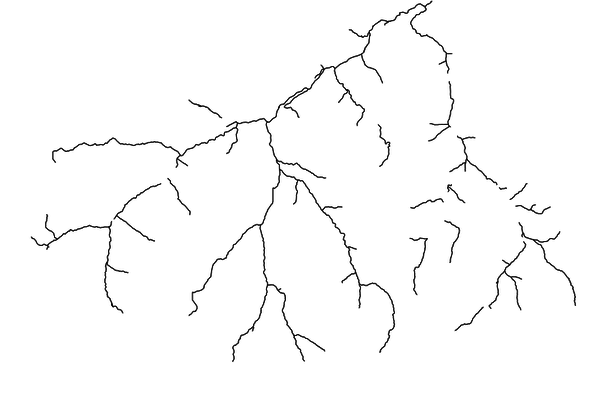input vector map availablestreams

First of all reset the region settings with g.region making them match the map elevation.

To create the map of this example, you can type in the following code in the command console or if you prefer you can only type in the main function names like r.green.hydro.recommended, d.vect or v.buffer in the console and specify the other parameters of the code like elevation or efficiency by using the graphical user interface.
```r.green.hydro.recommended              \
elevation=elevation                \
river=availablestreams             \
efficiency=0.9                     \
len_plant=200                      \
len_min=10                         \
distance=100                       \
output_plant=output_plant          \
discharge_current=currentdischarge \
mfd=mvf                            \
area=nationalparks                 \
buff=100                           \
p_min=20

d.vect map=output_plant color=blue

v.buffer input=nationalparks output=buff_park distance=100

d.vect map=buff_park7 color=0:128:0 fill_color=144:238:144 width=1
```

As you can see in the output map below, this code calculates the energy potential for a range of plant length from 10 to 200 m and a distance between the plants of 100 m. The areas with the national park and a buffer of 100 m around it are excluded. The discharge considered here is the current discharge of rivers reduced by 30% of the Minimum Flow Discharge.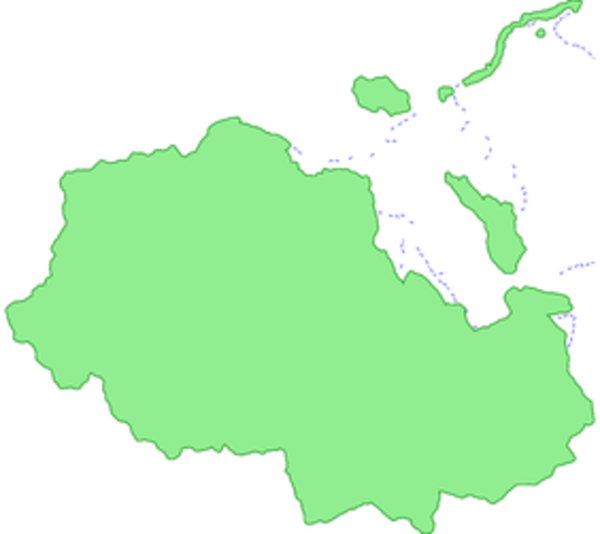output vector map: superimposition of the potential segments vector file (potentialplants, in blue) and the excluded national park (in green) and the buffer (in dark green)

EXAMPLE 2

The second example is based on the case-study of Mis valley in Belluno province, Veneto, Italy.

Here is the vector file availablestreams of the considered streams. The river segments already exploited by an existing plant do not appear in the file.
In superimposition, there are the vector map (in grey) of the national park we want to exclude and the points of interest (in green) used to create the visibility zones. These points were placed according to experts' recommendations during a focus group made in Veneto region.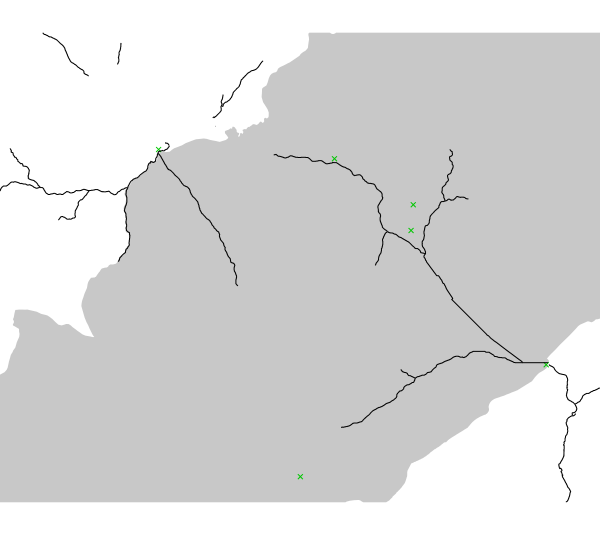input vector map availablestreams with the national park and points of interest

Points of interest are placed in the park so two different cases are presented here:
1) The national park and a buffer of 200 m around it are excluded
2) The visibility zones from points of interest is excluded

1) In the first case, the code used is:
```r.green.hydro.recommended              \
discharge_current=currentdischarge \
discharge_natural=naturaldischarge \
percentage=25.00                   \
river=availablestreams             \
elevation=elevation                \
efficiency=0.8                     \
len_plant=400                      \
len_min=10                         \
distance=150                       \
area=nationalparks                 \
buff=200                           \
output_plant=potentialplants

d.vect map=potentialplants color=blue

v.buffer input=nationalparks output=buff_park distance=200

d.vect map=buff_park color=255:179:179 fill_color=255:179:179 width=1
```

This command calculates the energy potential for a range of plant length from 10 to 400 m and a distance between plants of 150 m. The areas with the national park and a buffer of 200 m around it are excluded. The discharge considered here is the current discharge of rivers subtracted by 25% of the natural discharge (the latter corresponds to the MFD).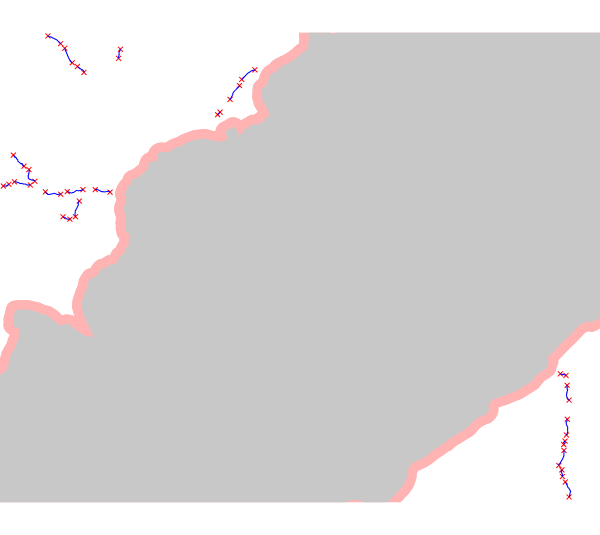output vector map: superimposition of the potential segments vector file (potentialplants, in blue), the excluded national park (in grey) and the buffer (in light red)

2) In the second case, the code used is:
```r.green.hydro.recommended                \
discharge_current=currentdischarge   \
mfd=mfd                              \
river=availablestreams               \
elevation=elevation                  \
efficiency=0.8                       \
len_plant=400                        \
len_min=10                           \
distance=150                         \
points_view=pointsinterest           \
n_points=1                           \
output_plant=potentialplants         \
output_vis=vis

d.vect map= potentialpoints color=red

d.vect map= potentialplants color=blue

d.vect map= pointsinterest color=green

d.vect map= vis color=144:224:144 fill_color=144:224:144 width=1
```

This command calculates the energy potential for a plant length range from 10 to 400 m and a distance between plants of 150 m. The visibility zones from each point of interest are excluded. The discharge considered here is the current discharge of rivers subtracted by the MFD. The MFD was calculated previously and computed in a raster map.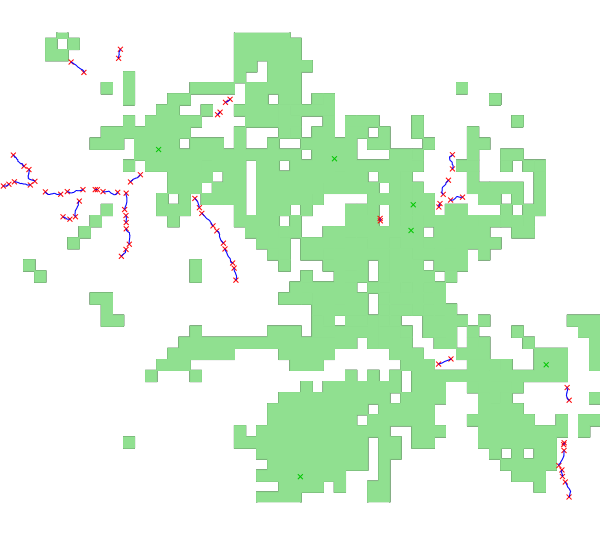output vector map: superimposition of the potential segments vector file (potentialplants, in blue), the points of interest (in green) and the visibility zones (in light green)

r.green.hydro.discharge
r.green.hydro.delplants
r.green.hydro.theoretical
r.green.hydro.optimal
r.green.hydro.structure
r.green.hydro.technical
r.green.hydro.financial

## AUTHORS

Giulia Garegnani (Eurac Research, Bolzano, Italy), Manual written by Julie Gros.

## SOURCE CODE

Available at: r.green.hydro.recommended source code (history)

Latest change: Mon Jun 28 07:54:09 2021 in commit: 1cfc0af029a35a5d6c7dae5ca7204d0eb85dbc55

Note: This addon document is for an older version of GRASS GIS that will be discontinued soon. You should upgrade your GRASS GIS installation, and read the current addon manual page.

© 2003-2023 GRASS Development Team, GRASS GIS 7.8.8dev Reference Manual